# Intersecting curves that don’t intersect

A post in MATLAB Answers earlier this year reminded me that working on a discrete grid can really mess up apparently obvious notions about geometry.

User Hg offered an image containing two intersecting curves. The problem is that the intersecting curves didn't intersect!

Here's the image:The red curve and the blue curve, which obviously cross each other, do not have any pixels in common. Hg's question: "What can I do to estimate the intersection point?"

Before I get into answering the original question, let me take a brief side trip to see if I can reconstruct the original input matrix (or at least something close). The image posted on MATLAB Answers had the pixels obviously blown up, with different colors applied to distinguish between the curves. Can I get back to just a matrix with 0s, 1s, and 2s corresponding to pixels belonging to the background and the two curves?

It helps that the image is a PNG file, which is losslessly compressed. I took a close look at the pixels using imtool, and I was able to determine that pixels belong to one curve were colored using [237 28 36], and pixels belonging to the second curve were colored using [0 162 232]. Also, each pixel seemed to be magnified by about a factor of 16. Let's run with those numbers.

url = 'https://www.mathworks.com/matlabcentral/answers/uploaded_files/44249/intersect2.png';
red = rgb(:,:,1);
green = rgb(:,:,2);
blue = rgb(:,:,3);

curve1 = (red == 237) & (green == 28) & (blue == 36);
curve2 = (red == 0) & (green == 162) & (blue == 232);
L = zeros(size(curve1));
L(curve1) = 1;
L(curve2) = 2;
imshow(L,[],'InitialMagnification','fit')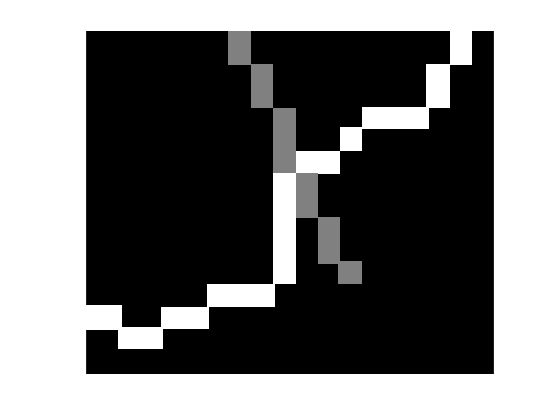That looks good. Let's try shrinking it down by a factor of 16.

L16 = imresize(L,1/16,'nearest');
imshow(L16,[],'InitialMagnification','fit');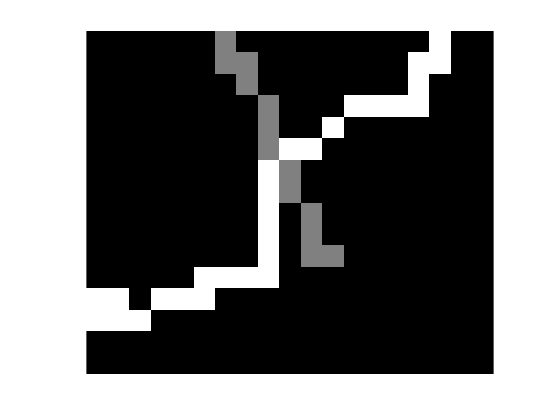Well, that's not perfect. But it's close enough to work with for answering Hg's question.

Image Analyst offered a potential solution:

There is no one pixel where the overlap occurs. If you'll accept any of those 4 pixel locations as an overlap, then perhaps if you dilated, ANDed, then called bwulterode. Something like (untested)

intImage = imdilate(bw1, true(3)) & imdilate(bw2, true(3));
intPoints = bwulterode(intImage);

Image Analyst's idea is to use morphological dilation to thicken each curve individually, then use a logical AND operator (&) to determine the set of pixels that belong to both thickened curves, and then compute the ultimate erosion using bwerode to shrink the intersection area down. Here it is in several steps.

curve1 = (L16 == 1);
curve2 = (L16 == 2);
curve1_thickened = imdilate(curve1,ones(3,3));
imshow(curve1_thickened,'InitialMagnification','fit')
title('Curve 1 (thickened)')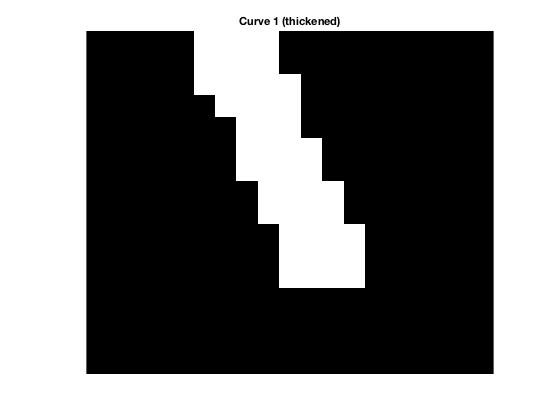curve2_thickened = imdilate(curve2,ones(3,3));
imshow(curve2_thickened,'InitialMagnification','fit')
title('Curve 2 (thickened)')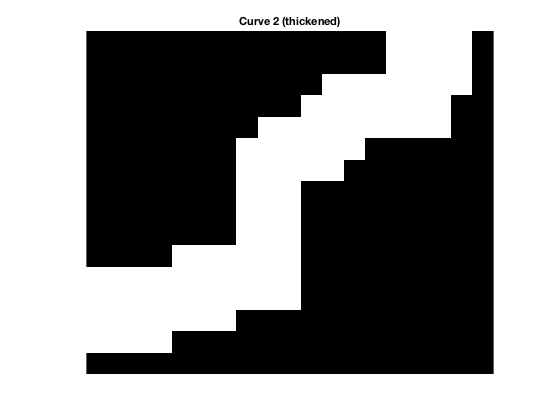curve_intersection = curve1_thickened & curve2_thickened;
imshow(curve_intersection,'InitialMagnification','fit')
title('Intersection of thickened curves')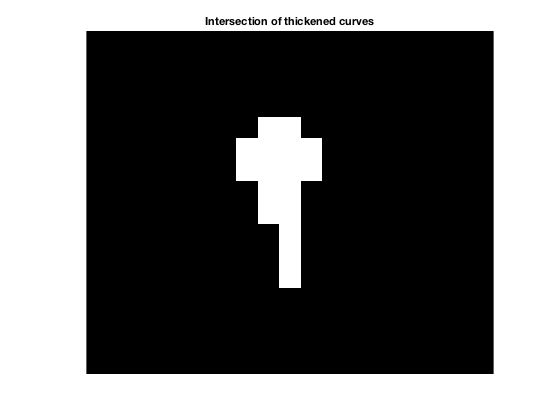ultimate_erosion = bwulterode(curve_intersection);
imshow(ultimate_erosion,'InitialMagnification','fit')
title('Ultimate erosion of intersection')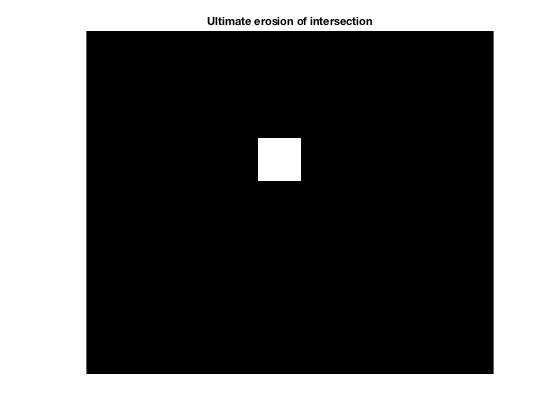Let's overlay the output of the ultimate erosion on top of the original image.

imshow(imfuse(L16,ultimate_erosion,'falsecolor'),'InitialMagnification','fit')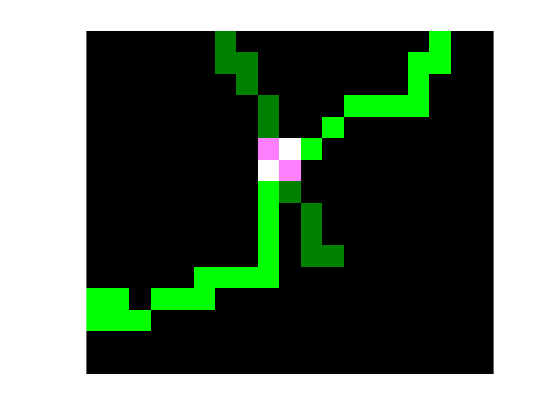You can see that the ultimate erosion is not a single point. An alternative is to compute the centroids of the intersection "blobs."

s = regionprops(ultimate_erosion,'Centroid')

s =

Centroid: [9.5000 6.5000]



There's just one intersection blob, and its centroid is at (9.5,6.5). Let's plot that.

imshow(L16,[],'InitialMagnification','fit')
hold on
plot(s.Centroid(1),s.Centroid(2),'go','MarkerSize',15,...
'MarkerFaceColor','g')
hold off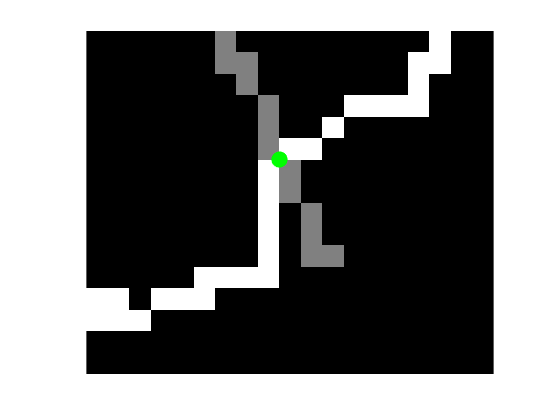How would you approach this problem? Leave your ideas and thoughts in the comments.

Published with MATLAB® R2016a

|